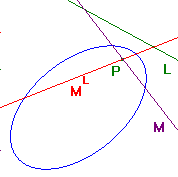the congugate diameters theorem for plane conics

 A diameter of a central conic is a line through the centre. We shall see that each diameter can be associated with another, known as its conjugate. This can be done with affine methods, but we would need to consider ellipses and hyperbolas separately. By working in projective geometry, we get a unified approach. We get a central conic by taking a p-conic C and a p-line L which is not a tangent to C. If we embed C on a plane Π parallel to L, then C(Π) is a central conic. The centre is the embedding of the p-point P, the pole of L with respect to C. At one or two points, we will need the following Lemma Suppose that C is a p-conic, and P is a p-point. Then P lies on its polar with respect to C if and only if P is on C. Note that, if a p-point P lies on a p-conic C, then the polar of P is the tangent to C at P. For reasons which will become apparent, we must exclude tangents in the theory that follows. Definitions Suppose that C is a p-conic, and L a p-line not tangent to C. Let P be the pole of L with respect to C. (a) A p-line M is an L-diameter if P lies on M. (b) If M is an L-diameter, then the L-conjugate of M is the polar of the intersection of L and M. It is denoted by ML. There are problems if we allow L to be a tangent to C. Suppose that L is the tangent to C at P. Then P is the pole of L. Now let M be a p-line through P. If M = L, then the 'intersection' of L and M is not a p-point. Thus, the L-conjugate is not defined. If M ≠ L, then as M passes through P, L and M meet at P. The polar of P is L, so every M ≠ L has L-conjugate L. This is not a particularly useful concept. Basic results on L-conjugates Suppose that C is a p-conic, and L a p-line not tangent to C. Let P be the pole of L with respect to C. (1) If M is an L-diameter, then ML is an L-diameter. (2) If N = ML, then M = NL. (3) M = ML if and only if M and L meet on C. Note that M = ML occurs only if L, M meet on C. Now the p-line L meets a p-conic in at most two points. Since L is not a tangent, there are either no intersections or exactly two. In the latter case, since an L-diameter M must pass through P, there are precisely two L-diameters M with M = ML. By (2), the remaining L-diameters occur in pairs (M,N), with each the L-conjugate of the other. On the other hand, if L does not meet C, then all the L-diameters occur in pairs.the conjugate diameters theorem If M a diameter of a central plane conic then there is a diameter M' such that (a) M' bisects all chords parallel to M, and (b) M bisects all chords parallel to M'. Proof A plane conic may be taken as C(Π), where C is a p-conic. The plane conic will be central provided that L, the p-line represented by the plane parallel to Π, passing through O is not a tangent to C. To avoid a proliferation of notation, we shall not distinguish a line K on the plane, and the p-line which embeds as K. As in the proof of the parallel chords theorem, we see that the L-conjugate of M embeds as a diameter M' which bisects any chord parallel to M. This proves (a). Part (b) follows since M is the L-conjugate of M'. Note that, if the plane conic is a hyperbola, then an asymptote is a diameter. Each line parallel to an asymptote cuts the conic just once, so there are no chords parallel to an asymptote. In the above proof, if M is an asymptote, the method yields M' = M. The conditions are vacuous.